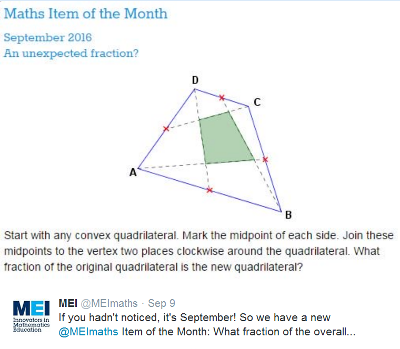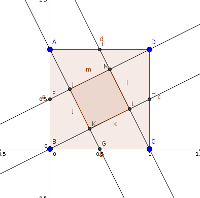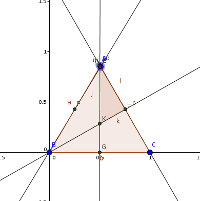An Unexpected FractionSubscribe!My latest posts can be found here:
Previous blog posts:

2016/09/18 - An Unexpected Fraction

On 2016-09-09, @MEIMaths tweeted an image and said:Quadrilateral Problem

 An Unexpected Fraction? Start with any convex quadrilateral. Mark the midpoint of each side, join these midpoints to the vertex two places clockwise around the quadrilateral. What fraction of the original quadrilateral is the new quadrilateral?

The way the question is phrased makes you feel two things.

Firstly, you have to feel that the answer is likely to be surprising in some way, so that means it's likely to be a nice puzzle.

But secondly, it seems clear from the phrasing that the answer must be the same regardless of the particular quadrilateral chosen.

Well, I've seen this before in the context of a square, and the answer there is lovely - it's a fifth. The proof in the case of a square is fairly simple, and there are a number of ways of going about it. So there we are, it's sorted, the answer is a fifth.

Nice one.Quadrilateral Problem The Square case
But then Hugh Hunt sent me an email saying:

 "In the limiting case of an equilateral triangle the answer is a sixth. "Am I doing something wrong?"Quadrilateral Problem Limiting Triangle
That's a good question. If we take two adjacent vertices of the square and move them together, just before they meet we do still have a convex quadrilateral, so because we expect the answer to be constant (because of the context) we would expect the answer still to be a fifth, but the answer is now pretty obviously a sixth.

So what's going on?

The short answer seems to be that @MEIMaths mis-spoke themselves, and despite the wording, they didn't actually mean any quadrilateral. But what might they have meant, and what answers might be possible?

So here are some interesting questions:

• Under what circumstances is the answer one fifth?

• Is the answer always between a sixth and a fifth?

• If not, what are the extreme values?

• Is every value in the range $[\frac{1}{6},\frac{1}{5}]$ achievable?

So even if it was a mistake, there is interesting mathematics to be found. That, at least, is no surprise.References

Here's the original tweet:

Adam Atkinson has created a GeoGebra twitchet you can play with:

 <<<< Prev <<<< You Have To Admire Their Optimism : >>>> Next >>>> Surprisingly Quick ...

 You should follow me on twitterI've decided no longer to include comments directly via the Disqus (or any other) system. Instead, I'd be more than delighted to get emails from people who wish to make comments or engage in discussion. Comments will then be integrated into the page as and when they are appropriate.

If the number of emails/comments gets too large to handle then I might return to a semi-automated system. That's looking increasingly unlikely.

ContentsSuggest a change ( <-- What does this mean?) / Send me email